• 文章目录1、超平面一般表示形式2、超平面的法向量3、点到超平面的距离4、平行超平面之间的距离公式   1、超平面一般表示形式 在n维空间中，设任意点坐标为 xT=[x(1),x(2),...x(n)]T∈Rnx^T=[x^{(1)},x^{(2)},...x^...
文章目录1、超平面一般表示形式2、超平面的法向量3、点到超平面的距离4、平行超平面之间的距离公式

1、超平面一般表示形式
在n维空间中，设任意点坐标为
$x=[x^{(1)},x^{(2)},...x^{(n)}]^T\in{R^n}$
设超平面参数
$w=[w^{(1)},w^{(2)},...w^{(n)}]^T\in{R^n}$
$b\in{R}$
则超平面方程可表示为
$w^T x+b=0\tag{1}$

2、超平面的法向量
超平面的法向量满足：超平面中任意向量都与该法向量垂直。设超平面上的两个点为$x_1$和$x_2$，分别满足：
$w^T x_1+b=0\tag{2}$
$w^T x_2+b=0\tag{3}$
两式相减，可得
$w^T (x_1-x_2)=0\tag{4}$
记 $\bm{v}=(x_1-x_2)$，由于$x_1$和$x_2$是任取的，故 $\bm{v}$ 表示超平面上的任意向量。这时我们可以发现，式$(4)$的含义恰好是：平面上任意一个向量都与 $w$ 相互垂直，因此 $w$ 就是超平面$w^T x+b=0$的一个法向量。

3、点到超平面的距离
记超平面外一点为 $x_0$ ，记点 $x_3$ 在超平面$w^T\cdot x+b=0$上的投影点为 $x_0'$，满足：
$w^T\cdot x_0'+b=0\tag{5}$
则有向量 $\bm{u}=(x_0-x_0')$ 与平面$w^T x+b=0$的法向量$\bm{w}$互相平行，则两者的数量积：
$w^T(x_0-x_0')=w\cdot (x_0-x_0')=|w|*|x_0-x_0'|*cos(0~or~\pi)=\pm|w|*d\tag{6}$
其中 $d=|x_0-x_0'|$ 即为待求的点到超平面间的距离。
另一方面，根据式$(5)$消去可得
$w^T(x_0-x_0')=w^Tx_0-w^Tx_0'=w^Tx_0-(-b)=w^Tx_0+b\tag{7}$
结合$(6)(7)$，考虑到 $d\ge0$，可得
$d=\frac{|w^Tx_0+b|}{|w|}\tag{8}$
这里上式中的 $|w|$ 表示 $w$ 的模长，模长作为绝对值概念的推广，在欧式空间中，模长常常称为L2范数（也称为Euclidean范数或者Frobenius范数）：
$||w||_F=\sqrt{(w^{(1)})^2+(w^{(2)})^2+...+(w^{(n)})^2}$
所以，$d$ 的表达式即为：
$d=\frac{|w^Tx_0+b|}{||w||_F}\tag{9}$
这样来看，平面直角坐标系下的点到直线距离公式便是上式的一个特例。

4、平行超平面之间的距离公式
趁热打铁，继续推导平行超平面间的距离公式，设两个不重合的平行超平面分别为：
$w_1^T x+b_1=0$
$w_2^T x+b_2=0$
由于两个超平面互相平行，因此由 $2$ 中对法向量的讨论可知，两个超平面的法向量互相平行，我们取两个互相重合的法向量，即
$w=w_1=w_2$
则可得
$w^T x+b_1=0\tag{10}$
$w^T x+b_2=0\tag{11}$
设 $P(x_0)$ 为平面1上的一个点，即满足：
$w^Tx_0+b_1=0\tag{12}$
则根据点到超平面的距离公式可得点 $P(x_0)$ 到超平面2的距离 $d$ 满足：
$d=\frac{|w^Tx_0+b_2|}{||x_0||_F}=\frac{|-b_1+b_2|}{||w||_F}$
上式最后一步用到了式$(12)$。最后我们得到了平行超平面之间的距离公式为
$d=\frac{|b_2-b_1|}{||w||_F}\tag{13}$


展开全文线性代数 矩阵 动态规划
• ## SVM：任意点到超平面的距离公式

万次阅读 多人点赞 2018-10-18 11:05:06
任意点 到超平面的距离公式 在样本空间中，划分超平面可通过... b 为位移项，决定了超平面与原点之间的距离.显然，划分超平面可被法向量 ω 和位移 b 确定 。 任意点到超平面的距离公式为： 推到如下： ...
任意点 到超平面的距离公式
在样本空间中，划分超平面可通过如下线性方程来描述:其中 w 决定了超平面的方向 ; b 为位移项，决定了超平面与原点之间的距离.显然，划分超平面可被法向量 ω 和位移 b 确定 。
任意点到超平面的距离公式为：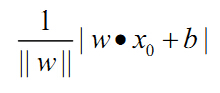推到如下：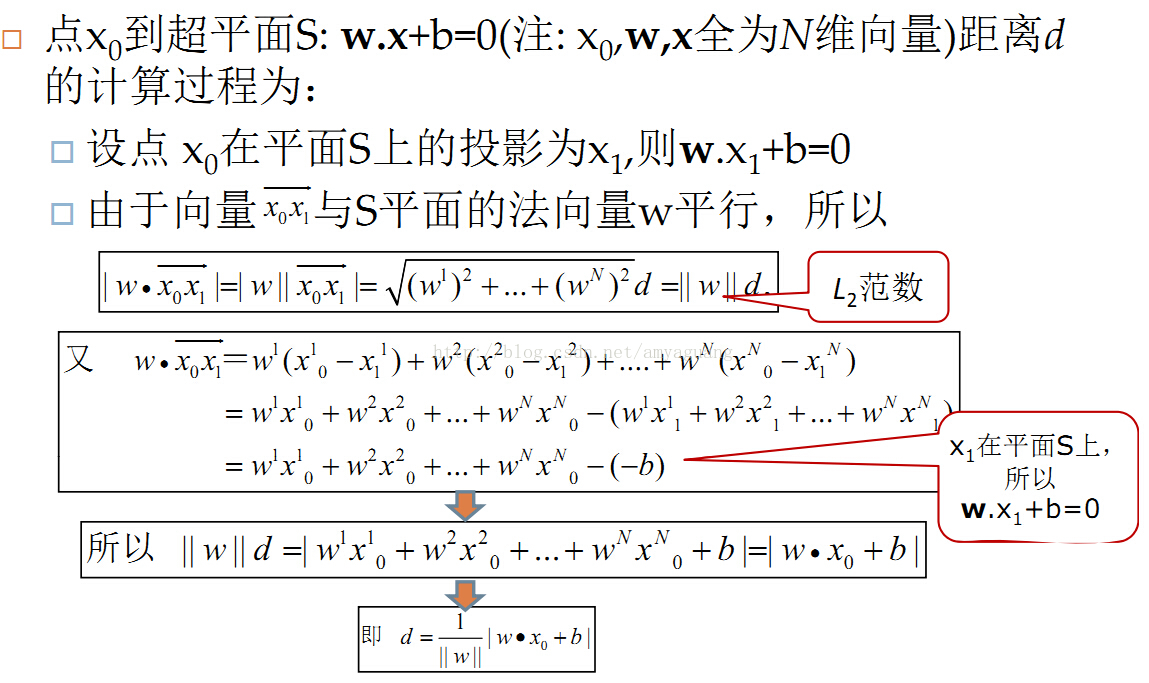展开全文• 其中，n=(A,B,C)是平面的法向量，D决定了平面与原点之间的距离，当D=0时，平面经过原点。   (2) 向量的模(长度)： 给定一个向量V=(x,y,z)，则   (3) 向量的点积(内积)： 给定两个向量和，则它们的内积为：...
预备知识：

(1) 平面的一般表达式：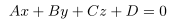其中，n=(A,B,C)是平面的法向量，D决定了平面与原点之间的距离，当D=0时，平面经过原点。

(2) 向量的模(长度)：

给定一个向量V=(x,y,z)，则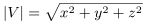(3) 向量的点积(内积)：

给定两个向量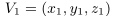和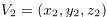，则它们的内积为：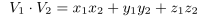点到直线的距离公式推导：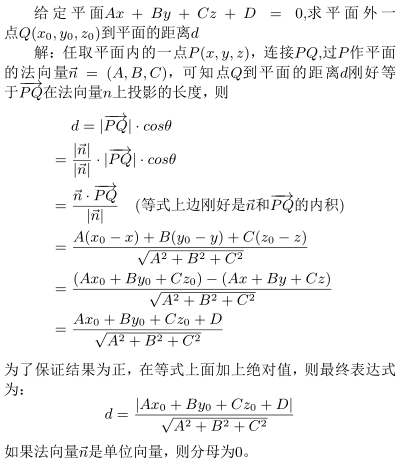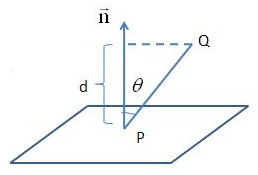参考博客：http://www.cnblogs.com/graphics/archive/2010/07/10/1774809.html

--------------------- 本文来自 懒懒的杰威 的CSDN 博客 ，全文地址请点击：https://blog.csdn.net/u011483307/article/details/51034169?utm_source=copy
展开全文• b 为位移项，决定了超平面与原点之间的距离.显然，划分超平面可被法向量 ω 和位移 b 确定 。 其中任意点到超平面的距离公式y为： 上述是点在超平面的正一侧的情形，如何点再在超平面负的一侧，只需在公式前加-...
支持向量机就是找到划分超平面中间隔最大的超平面，设定划分超平面的线性方程为：其中 w 决定了超平面的方向 ; b 为位移项，决定了超平面与原点之间的距离.显然，划分超平面可被法向量 ω 和位移 b 确定 。

其中任意点到超平面的距离公式y为：上述是点在超平面的正一侧的情形，如何点再在超平面负的一侧，只需在公式前加-号即可。

综上所述，点与超平面的距离公式可表示为：
yi*y

展开全文• 在样本空间中，划分超平面可通过如下线性方程来描述:wTx...b 为位移项，决定了超平面与原点之间的距离； 样本空间中，任意点x到超平面(w,b)的距离公式为： r=∣wTx+b∣∣∣w∣∣(西瓜书,6.2)r = \frac{|w^Tx+b|} {||...西瓜书
• 之间的距离 选证 点到空间直线的距离 点到平面的距离 异面直线之间的距离 点到点的距离 点到直线的距离 点到面的距离 直线直线间的距离 直线面的距离 面之间的距离 选证 点到...
• ## 数学中的常见的距离公式

万次阅读 多人点赞 2016-03-15 09:28:25
1. 欧氏距离，最常见的两点之间或多点之间的距离表示法，又称之为欧几里得度量，它定义于欧几里得空间中，如点 x = (x1,...,xn) 和 y = (y1,...,yn) 之间的距离为： (1)二维平面上两点a(x1,y1)b(x2,y2)间的...
• 中考热点(4) ——利用两点间的距离公式解决问题 中考25题是以函数为背景，在平面直角坐标系中,任意两点P1(x1,y1)、P2(x2,y2)，它们之间的距离为两点间的距离公式是数学中最基本的公式,它用代数方法解决了几何中的...
• 1. 欧氏距离，最常见的两点之间或多点之间的距离表示法，又称之为欧几里得度量，它定义于欧几里得空间中，如点 x = (x1,...,xn) 和 y = (y1,...,yn) 之间的距离为：(1)二维平面上两点a(x1,y1)b(x2,y2)间的欧氏距离...
• 概率分布之间的距离度量以及python实现 1. 欧氏距离(Euclidean Distance)  欧氏距离是最易于理解的一种距离计算方法，源自欧氏空间中两点间的距离公式。 (1)二维平面上两点a(x1,y1)b(x2,y2)间的欧氏距离...
• 求解距离矩阵的时候，我们通常会想到用欧氏距离公式直接求解两点之间的距离，但我们往往忽略了欧氏距离公式的使用前提是在平面上，而经纬度坐标是基于地球这一个球面的， 所以我们第一步应该将经纬度坐标转为直角...数学建模 线性代数
• 总结各类距离度量方法...  最常见的两点之间或多点之间的距离表示法，又称之为欧几里得度量，它定义于欧几里得空间中，如点 x = (x1,…,xn) 和 y = (y1,…,yn) 之间的距离为： (1)二维平面上两点a(x1,y1)b(...
• ## 各种距离的定义

千次阅读 2019-09-14 07:28:55
1、欧式距离（欧几里得距离） 欧式... 二维平面上点 之间的距离公式是： n维空间上点 和点 之间的距离公式是： 2、曼哈顿距离 曼哈顿距离是各坐标点的坐标差值相加。 二维平面上点 之间的距离公式...
• 焦距是从镜头的中心点到胶平面上所形成的清晰影像之间的距离。焦距的大小决定着视角的大小，焦距数值小，视角大，所观察的范围也大；焦距数值大，视角小，观察范围小。根据焦距能否调节，可分为定焦镜头和变焦镜头两...
• 有些时候我们在看计算机一些书籍的时候经常会涉及到空间中点之间的关系，书中会突然出现一些公式，可能很多时候我们会忘了证明的方法。 今天来谈谈点到空间中面的公式。 下面先给出空间中面的表示公式： w...
• 面面、线面、线线之间的夹角，点到直线的距离以及点到平面的距离，求平面方程和直线方程，利用平面、直线的相互关系(平行、垂直、相交等)解决有关问题。下面的★表示该题的难度系数。一、填空题(每题3分，共18分)二...
• 欧氏距离最常见的两点之间或多点之间的距离表示法，又称之为欧几里得度量，它定义于欧几里得空间中。 二维平面上两点a(x1,y1)，b(x2,y2)之间的欧式距离公式： 二.曼哈顿距离我们可以定义曼哈顿距离的正式意义为L1-...
• 整理下最近回顾相似度计算的笔记 一、欧式距离 欧式距离是最常见的一种距离度量方式，衡量的是多维空间中两个...2、二维平面上两点a(x1,y1)b(x2,y2)间的欧氏距离： 3、两个n维向量和向量之间的欧式距离为： ...
• 欧氏距离Euclidean distance:欧几里得距离，m维空间中两个点之间的真实距离离差平方和，开根号，得到结果二维平面上点a(x1,y1)b(x2,y2)间的欧氏距离不一定需要进行中心化和标准化公式表示：标准化欧氏距离 ...马氏距离计算实例
• 欧氏距离Euclidean distance:欧几里得距离，m维空间中两个点之间的真实距离离差平方和，开根号，得到结果二维平面上点a(x1,y1)b(x2,y2)间的欧氏距离不一定需要进行中心化和标准化公式表示：标准化欧氏距离 ...
• 1. 欧氏距离，最常见的两点之间或多点之间的距离表示法，又称之为欧几里得度量，它定义于欧几里得空间中，如点 x = (x1,...,xn) 和 y = (y1,...,yn) 之间的距离为： (1)二维平面上两点a(x1,y1)b(x2,y2)间的欧氏...
• 简要地叙述了厄米高斯模拉盖尔高斯模的一些理论，画出了具有代表性的厄米高斯模拉盖尔高斯模在z=0平面的三维...画出横截面中振幅强度随传输距离增加而变化的图像，从中得到了束宽和峰值强度传输距离之间的关系。
• 根据投影中心投影平面（观察平面之间的距离（或者说，投影线是否相交于一点，或者说是否保持对象的比例），分为平行投影 和 透视投影。 平行投影 根据投影方向（视线方向）投影平面的夹角是否垂直，平行...计算机视觉
• A为电源平面与地平面重叠部分的面积：d为电源平面与平面之间的距离。 　显然，用电磁场的方法来分析电源／地平面对的谐振特性是最好不过的，但困难在于建模复杂，计算量大，对分析人员要求的电磁场理论知识更高，...
• 3·3 各种平均值之间的关系 3·4 累积金和分期付款 4.各种数列的和 4·1 乘幂数列的和 4·2 差分数列 4·3 通项是n的整式的数列 4·4 分数项数列 4·5 Σanxn(an是等差数列) 4·6 二重数列相似形 5.数学归纳法 5·...
• 3·3 各种平均值之间的关系 3·4 累积金和分期付款 4.各种数列的和 4·1 乘幂数列的和 4·2 差分数列 4·3 通项是n的整式的数列 4·4 分数项数列 4·5 Σanxn(an是等差数列) 4·6 二重数列相似形 5.数学归纳法 5·...
• 物距就是指物体到透镜光心的距离，像距是像到平面镜（或透镜的光心）之间的距离。 对焦即是改变镜头光心底片ccd(或cmos)的距离，实现图像清晰地显示出来，变焦利用凹透镜凸透镜的叠加，通过调整相互的...
• 从中国象棋的角度，理解支持向量机【印象深刻...边缘的定义是决策边界任意样本之间的最⼩距离！ 支持向量机的工作核心是：找分界平面，N-1维的超平面，最大化边缘，也就是最小距离最大化。 公式推导。 拉普拉斯算子。机器学习 svm 大数据 python